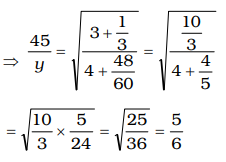## Speed, Time and Distance

#### Speed, Time and Distance

1. Two trains, A and B, start from stations X and Y towards Y and X respectively. After passing each other, they take 4 hours 48 minutes and 3 hours 20 minutes to reach Y and X respectively. If train A is moving at 45 km/hr., then the speed of the train B is
1. 60 km/hr
2. 64.8 km/hr
3. 54 km/hr
4. 37.5 km/hr

1. Let speed of train A = x kmph
and speed of train B = y kmph

 ∴ x = √ t2 y t1⇒ 5y = 45 × 6 ⇒ y = 45 × 6 = 54 kmph 5

##### Correct Option: C

Let speed of train A = x kmph
and speed of train B = y kmph

 ∴ x = √ t2 y t1⇒ 5y = 45 × 6 ⇒ y = 45 × 6 = 54 kmph 5

1. A is twice as fast runner as B, and B is thrice as fast runner as C. If C travelled a distance in 1 hour 54 minutes, the time taken by B to cover the same distance is
1. 19 minutes
2. 38 minutes
3. 51 minutes
4. 57 minutes

1. Ratio of the speed of A and B
= A : B = 2 : 1 = 6 : 3
B : C = 3 : 1
∴  A : B : C = 6 : 3 : 1
∴  Ratio of their time taken

 = 1 : 1 : 1 = 1 : 2 : 6 6 3

 ∴  Time taken by B =2 × 114minutes 6

= 38 minutes

##### Correct Option: B

Ratio of the speed of A and B
= A : B = 2 : 1 = 6 : 3
B : C = 3 : 1
∴  A : B : C = 6 : 3 : 1
∴  Ratio of their time taken

 = 1 : 1 : 1 = 1 : 2 : 6 6 3

 ∴  Time taken by B =2 × 114minutes 6

= 38 minutes

1. Ravi travels 300 km partly by train and partly by car. He takes 4 hours to reach, if he travels 60 km by train and rest by car. He will take 10 minutes more if he were to travel 100 km by train and rest by car. The speed of the train is :
1. 50 km/hr
2. 60 km/hr
3. 100 km/hr
4. 120 km/hr

1. Let the speed of train be x kmph and that of car be y kmph,
then

 60 + 240 = 4   ...(i) x y

 and, 100 + 200 = 25 x y 6

 ⇒ 4 + 8 = 1 ...(ii) x y 6

By equation (i ) – equation (ii) × 30
 60 + 240 − 120 − 240 = 4 – 5 x y x y

 ⇒  − 60 = − 1 x

⇒  x = 60 kmph

##### Correct Option: B

Let the speed of train be x kmph and that of car be y kmph,
then

 60 + 240 = 4   ...(i) x y

 and, 100 + 200 = 25 x y 6

 ⇒ 4 + 8 = 1 ...(ii) x y 6

By equation (i ) – equation (ii) × 30
 60 + 240 − 120 − 240 = 4 – 5 x y x y

 ⇒  − 60 = − 1 x

⇒  x = 60 kmph

1. A man has to be at a certain place at a certain time. He finds that he shall be 20 minutes late if he walks at 3 km/hour speed and 10 minutes earlier if he walks at a speed of 4 km/hour . The distance he has to walk is
1. 24 km
2. 12·5 km
3. 10 km
4. 6 km

1. Let the required distance be x km.

 ∴ x − x = 30 3 4 60

 ⇒ x = 1 ⇒ x = 1 × 12 = 6 km 12 2 2

##### Correct Option: D

Let the required distance be x km.

 ∴ x − x = 30 3 4 60

 ⇒ x = 1 ⇒ x = 1 × 12 = 6 km 12 2 2

1. If I walk at 5 km/hour, I miss a train by 7 minutes. If, however, I walk at 6 km/hour, I reach the station 5 minutes before the departure of the train. The distance (in km) between my house and the station is
1. 6
2. 5
3. 4
4. 3

1. Let the required distance be x km, then

 x − x = 12 = 1 5 6 60 5

 ⇒ 6x − 5x = 1 ⇒ x = 1 30 5 30 5

⇒  x = 6 km.

##### Correct Option: A

Let the required distance be x km, then

 x − x = 12 = 1 5 6 60 5

 ⇒ 6x − 5x = 1 ⇒ x = 1 30 5 30 5

⇒  x = 6 km.